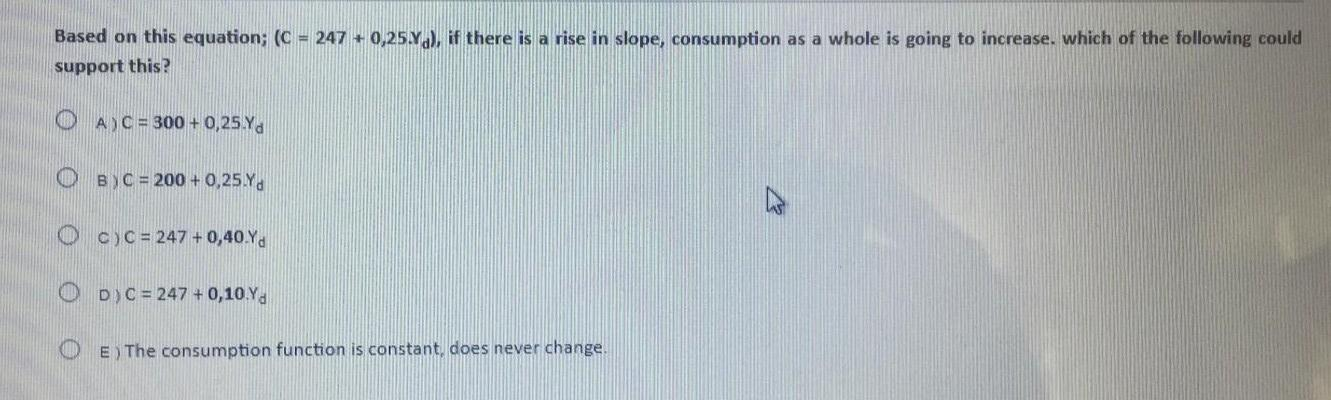### Create an Account

Already have account?

### Forgot Your Password ?

Home / Questions / Based on this equation; (C = 247 +0,25%.), if there is a rise in slope, consumption as a w...

# Based on this equation; (C = 247 +0,25%.), if there is a rise in slope, consumption as a whole is going to increase. which of the following could support this? O A) C = 300 + 0,25Yd OBC = 200 + 0,25 Y

Based on this equation; (C = 247 +0,25%.), if there is a rise in slope, consumption as a whole is going to increase. which of the following could support this? O A) C = 300 + 0,25Yd OBC = 200 + 0,25 Ya Oc) C = 247 +0,40.Yd OD) C = 247 + 0,10.Yd O E) The consumption function is constant, does never change.Apr 15 2021 View more View Less

#### Answer (Solved)Subscribe To Get Solution